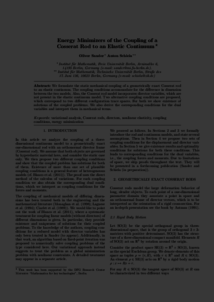Repository: Freie Universität Berlin, Math Department

# Energy minimizers of the coupling of a Cosserat rod to an elastic continuum

Sander, O. and Schiela, A. (2012) Energy minimizers of the coupling of a Cosserat rod to an elastic continuum. Mathematical Modelling, 7 (1). pp. 1118-1123.Preview

295kB

## Abstract

We formulate the static mechanical coupling of a geometrically exact Cosserat rod to an elastic continuum. The coupling conditions accommodate for the diﬀerence in dimension between the two models. Also, the Cosserat rod model incorporates director variables, which are not present in the elastic continuum model. Two alternative coupling conditions are proposed, which correspond to two diﬀerent conﬁguration trace spaces. For both we show existence of solutions of the coupled problems. We also derive the corresponding conditions for the dual variables and interpret them in mechanical terms.

Item Type: Article Mathematical and Computer Sciences > Mathematics > Numerical Analysis Department of Mathematics and Computer Science > Institute of Mathematics 1870 Ekaterina Engel 30 Mar 2016 17:49 03 Mar 2017 14:42

Repository Staff Only: item control page